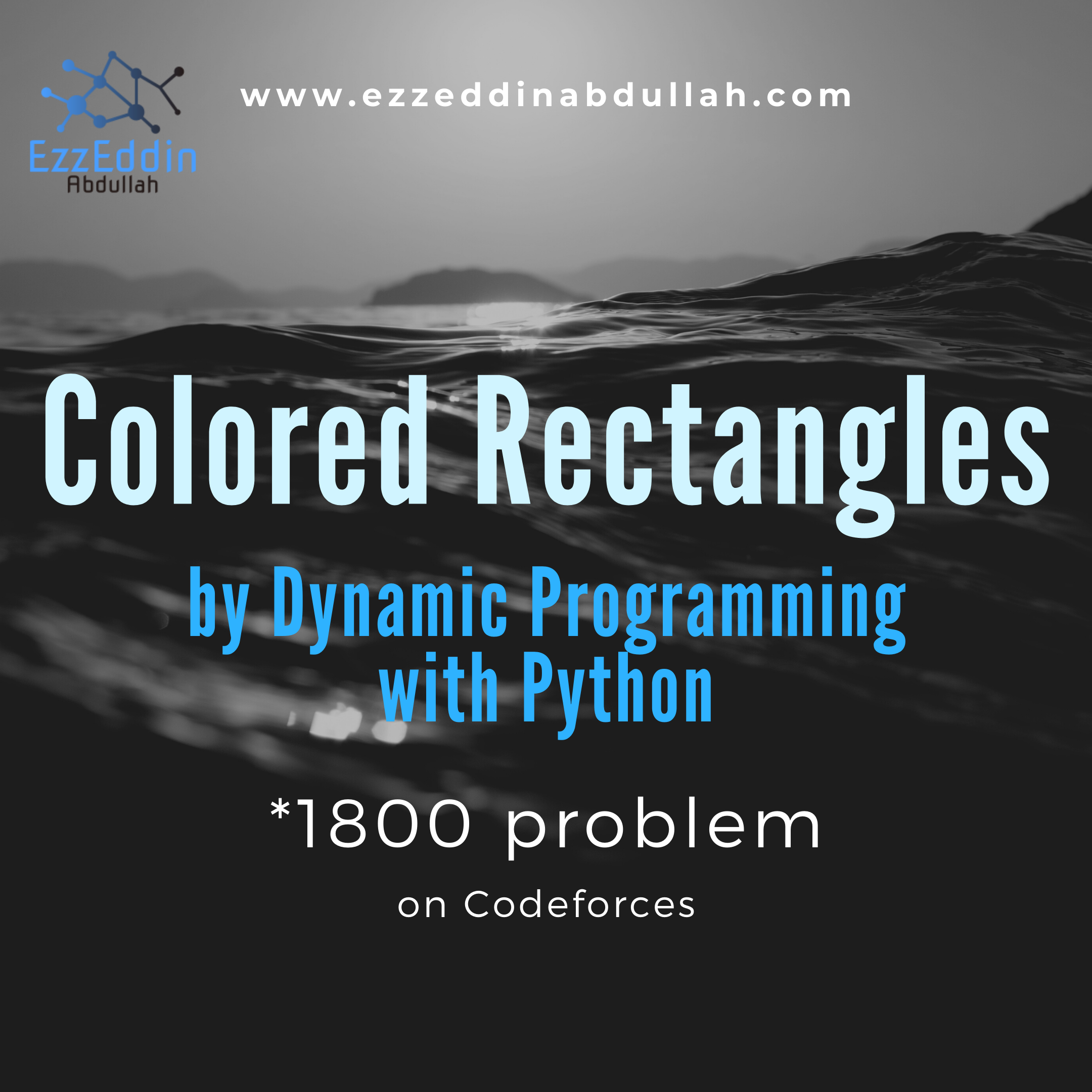# Colored Rectangles by Dynamic Programming with Python

Using Dynamic Programming with Python solution: Let's learn how to think about Colored Rectangles, an 1800-point codeforces problem## Understanding the Problem

Colored Rectangles is an 1800-point problem at codeforces, I hope you have read the problem carefully and have tried solving it. This problem basically requires to get the largest area of colored rectangles. The question is how to get that large area and what givens we have in this problem.

You’re given pairs of colored sticks (red, green, and blue) and you know the length of each pair. To compute the area of that rectangle you have to multiply the length with the width. Say we have one pair of length 3 (which means 2 parallel sides of length 3) and another pair of length 4 (which means 2 parallel sides of length 4) so they can form a rectangle whose area is 12.

## Inputs

This problem has four inputs:

• R, G, and B which are the numbers of red, blue, and green pairs of sticks respectively
• Lengths of the red pairs of sticks
• Lengths of the blue pairs of sticks
• Lengths of the green pairs of sticks

## Why done by Dynamic Programming?

This problem seems trivial at first so if you have 3, 4, 5 as lengths of red, blue, and green pairs of sticks. It seems we should compute the area for each possible combination and then compare between them to see the maximum area which is 20 (4*5). But what if we have the same set of pairs plus 6 pairs of green sticks meaning we have:

• rl = 
• gl = [4, 6]
• bl = 

where each of rl, gl, bl corresponds to the list of red, blue, and green sticks respectively

In this case, we should care about the order of selecting the numbers we choose to calculate the area which means selecting the highest numbers first which implies sorting each array so that we can multiply the biggest numbers first. So solving that will get the best area to be 42 (which is 6 * 5 + 3 * 4)

This problem can be tricky, so life is not as smooth as in the last examples. We can meet such numbers:

• rl = 
• gl = [2, 2]
• bl = 

This means if we apply what we did in the last example, we get 9 (which is 3 * 3) and that’s because 3 and 3 are the biggest numbers while if we consider multiplying 3 by 2 and then 2 by 3 we get 12 which is obviously a bigger area. In this case, we should consider a way to store the products of each combination and make a decision on calculating the best area.

This can be done by dynamic programming, we need first to form a 3D array to store the areas we computed to be able to compare them.

This problem can be more trickier if we have:

• rl = [10, 3, 2]
• gl = [8, 6, 4]
• bl = [8, 6, 2]

I have already sorted each array in descending order. Imagine these are stacks and each element you multiply is popped out of it (removed). The following is a suggested way to do the multiplications:

1. 10 * 8 (from rl and bl)
2. 8 * 6 (from bl and gl)
3. 6 * 3 (from gl and rl)
4. 2 * 4 (from rl and gl)

If you compute the sum of these areas you’ll get 154 but this is not the biggest area so this suggested solution is wrong. Let’s see another suggested multiplications:

1. 10 * 8 (from rl and gl)
2. 8 * 6 (from bl and gl)
3. 6 * 4 (from bl and gl)
4. 3 * 2 (from rl and bl)

This combination gives us the biggest area which is the right answer. If you notice the difference, you can see that gl has [8, 6, 4] and bl has [8, 6, 2]. It looks like we have equal numbers here so the question here how we can decide which number will be multiplied first. Should we multiply what’s in rl with gl first or rl with bl. Comparing the two suggestion solutions, the first 3 steps give us a bigger number. The total area for the second solution is 158 which is bigger than the first one. This happened because we want to leave off the smallest number at the end which exists in bl array. That’s why we took what’s in rl and gl first before we can reach the end of the blue array. This ensures that we need a way to recursively compute the area at each node so that we can decide which path we should choose.

The problem with the recursive solution is the computation time, so we can optimize this by saving the states instead of computing the area again at each state so that we can reuse this information on the area we have and then decide if it’s the best or not.

That’s how I thought about this problem and below is an implementation in Python submitted by srkvrm:

## Other problem-solving stuff

If you want to see more problem-solving blog posts, check out:

https://www.ezzeddinabdullah.com/category/problem-solving

# Join the conversation